13Motion of a charged particle in the magnetic field
Оценка 4.8

# 13Motion of a charged particle in the magnetic field

Оценка 4.8
docx
07.05.2020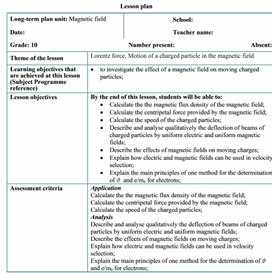13Motion of a charged particle in the magnetic field.docx

Lesson plan

 Long-term plan unit: Magnetic field School: Date: Teacher name: Grade: 10 Number present: Absent: Theme of the lesson Lorentz force. Motion of a charged particle in the magnetic field Learning objectives that are achieved at this lesson (Subject Programme reference) ·         to investigate the effect of a magnetic field on moving charged particles; Lesson objectives By the end of this lesson, students will be able to: ·         Calculate the the magnetic flux density of the magnetic field; ·         Calculate the centripetal force provided by the magnetic field; ·         Calculate the speed of the charged particles; ·         Describe and analyse qualitatively the deflection of beams of charged particles by uniform electric and uniform magnetic fields; ·         Describe the effects of magnetic fields on moving charges; ·         Explain how electric and magnetic fields can be used in velocity selection; ·         Explain the main principles of one method for the determination of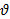and e/me for electrons; Assessment criteria Application Calculate the the magnetic flux density of the magnetic field; Calculate the centripetal force provided by the magnetic field; Calculate the speed of the charged particles; Analysis Describe and analyse qualitatively the deflection of beams of charged particles by uniform electric and uniform magnetic fields; Describe the effects of magnetic fields on moving charges; Explain how electric and magnetic fields can be used in velocity selection; Explain the main principles of one method for the determination ofand e/me for electrons; Language objectives Subject-specific vocabulary & terminology magnetic force on a moving charge a beam of electrons electron gun Fleming’s left-hand rule deflected up the page deflected down the page into the page out of page the magnetic flux density B (strength of the magnetic field) the charge Q on the particle the speed v of the particle Useful set(s) of phrases for dialogue/writing A charged particle entering at right angles to a uniform magnetic field describes a circular path because the magnetic force is perpendicular to the velocity. Type of differentiation Differentiated poster-session , Collaborative Learning, Progressive Task with Digital resources Values instilled at the lesson Safety, Consideration to others, Co-operation, Opportunity for Life-Long Learning, Academic Integrity and Transparency, Respect for Self and Others Cross-curricular links Mathematics, Chemistry ICT skills Research skills, use of video as introduction Previous learning Grade 8: magnetic fields; representation of fields by field lines; fields of permanent magnets Grade 8: electrical equations: V = IR, P = IV Course of the lesson Planned stages of the lesson Planned activities at the lesson Resources Beginning (0-3 min)                     (4-7 min) Teacher: -Introduces the topic of day and spelling out the learning outcome they will possess after the study. 1. Organizational moment to acquaint students with the ·         The theme of the lesson ·         The objectives of the lesson ·         The criteria of success for the lesson ·         The plan of events for the lesson     Motivation. Thompson’s experiment Thomson’s role in the discovery of the electron, it leads learners to be interested to study of the new material. Ø  In 1897 JJ Thomson set out to prove that cathode rays originating from a heated cathode (electron gun), were actually a stream of small negatively charged particles called electrons. Ø  Electrons are accelerated from the cathode. Ø  They are deflected by electric and magnetic fields. Ø  The beam of electrons strikes a fluorescent screen. Ø  e/m was measured. Ø  Today we will learn more together about it! Middle 8-14 min     15-19 min      20-26 min (W) Whole class work. The study of the new material is based on the explanation of the topic by the teacher accompanied by a presentation.   (D) Video demonstration. Use the fine beam tube video to derive a value for e/m.     Discussion: Velocity selectors and mass spectrometers There is a wide range of these both of working principles. Again there is a chance to compare the effects of electric and magnetic fields. If students are studying chemistry, you can draw on their knowledge of mass spectrometers to emphasise the value of these techniques. 27-37 min (f) Formative assessment. A set of calculations based on the velocity selector can be used to test understanding of the underlying principles and use of equations.             Write your answers in the spaces provided. Use these data: ·         magnitude of the electronic charge = 1.6 × 10–19 C ·         mass of electron = 9.1 × 10–31 kg The force on an electric current in a magnetic field is perpendicular to both the current and the field. This diagram may help you.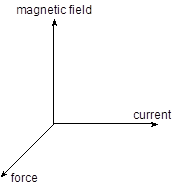Questions A beam of particles passes in a straight line from a source X, to a spot, E, on a fluorescent screen. When the electromagnet shown is switched on, the beam hits the screen at one of the spots A, B, C, D or E.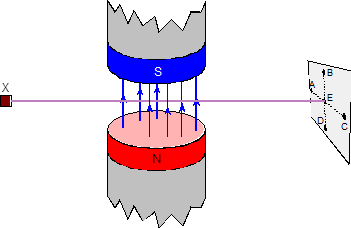1.         In which direction does the beam bend if the particles are positively charged? 2.         Where does it go if the particles are neutral? 3.         What happens to the positively charged particles if the magnetic field is reversed? In one form of mass spectrometer, charged ions in the beam fan out, moving in the paths shown in the diagram. Parts of the paths include a magnetic field whose direction is perpendicular to the plane of the paper.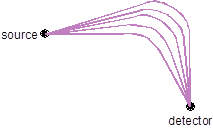4.         Indicate places where there must be no magnetic field in the direction suggested. Do not   use shading. 5.         Shade the area where there must be a magnetic field. 6.         What is the shape of the path followed by a proton that is projected into a uniform             magnetic field at right angles to its velocity? Justify your answer.   The diagram shows the initial path of an electron fired into a uniform magnetic field. The magnetic field is at right angles to the direction of the electron and is directed away from the reader.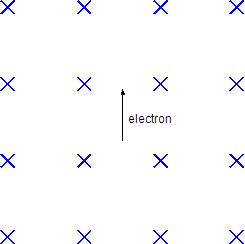7.         Add a labelled arrow to the show the direction of the force on the electron. 8.         Draw the subsequent path of the electron. A uniform electric field is produced by maintaining a potential difference of 1000 V across a pair of parallel plates 5 cm apart. An electron enters the field at right angles as shown with a velocity of 4.0  107 m s–1 and emerges from the plates without hitting them.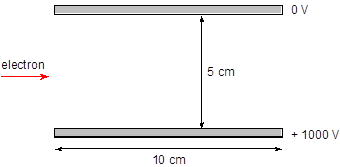9.         Draw in the path of the electron on the diagram. 10.       What name is given to this type of path?   Hints 1.         Remember that the left-hand motor rule works for conventional current. 2.         Remember that the left-hand motor rule works for conventional current. Ending (38-40 min) At the end of the lesson, learners reflect on their learning: -           What has been learned -           What remained unclear -           What is necessary to work on Where possible the learners could evaluate their own work as well as the work of their classmates using certain criteria. Differentiation – how do you plan to give more support? How do you plan to challenge the more able learners? Assessment – how are you planning to check students’ learning? Health and safety regulations ·         Multiple Intelligences -          Visual will watch the video -          Analytical take information from the texts   ·         Differentiation by questioning and dividing in group ·         Worksheet with varied difficulties Assessment – how are you planning to check students’ learning? The output for the worksheet will serve as assessment Questions during the lesson will also serve as formative assessment. Be careful when use the laser-coder Reflection   Were the lesson objectives/learning objectives realistic? Did all learners achieve the LO? If not, why? Did my planned differentiation work well? Did I stick to timings? What changes did I make from my plan and why? Use the space below to reflect on your lesson. Answer the most relevant questions from the box on the left about your lesson. Summary evaluation     What two things went really well (consider both teaching and learning)? 1:   2:   What two things would have improved the lesson (consider both teaching and learning)? 1:   2:   What have I learned from this lesson about the class or achievements/difficulties of individuals that will inform my next lesson?

## Type of differentiation Differentiated poster-session ,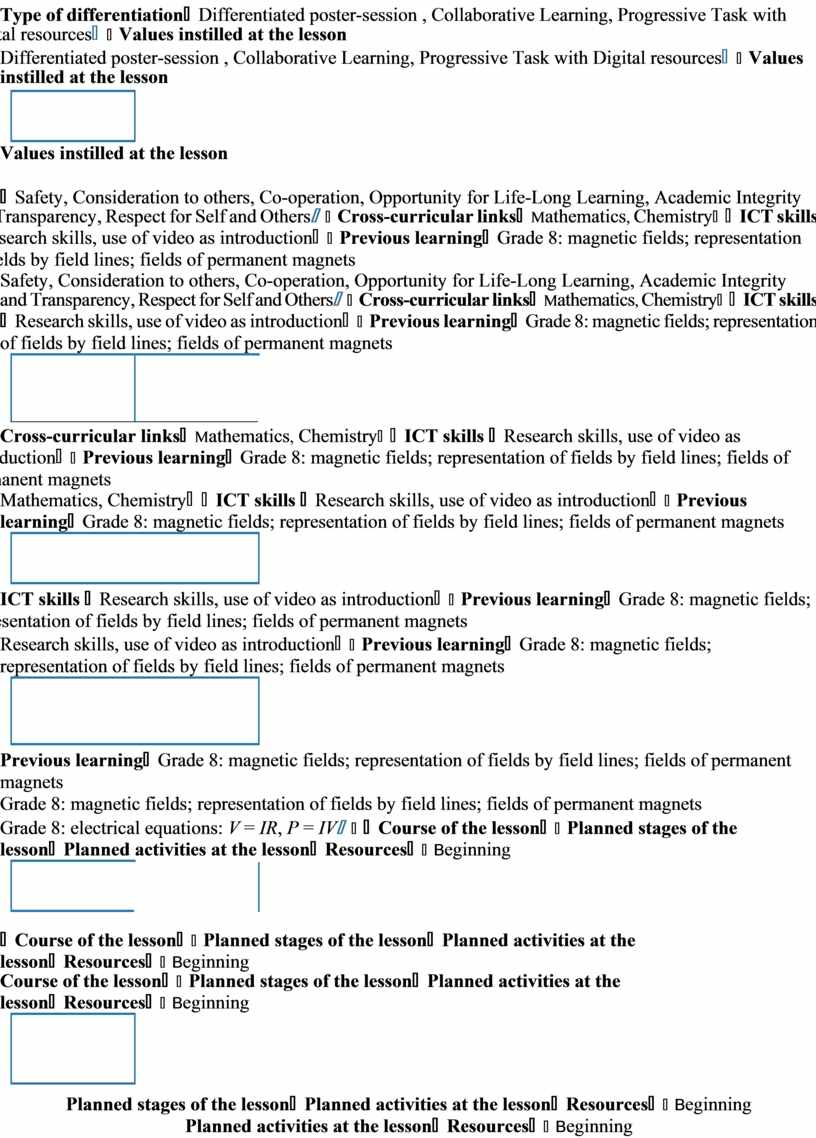## W) Whole class work. The study of the new material is based on the explanation of the topic by the teacher accompanied by a presentation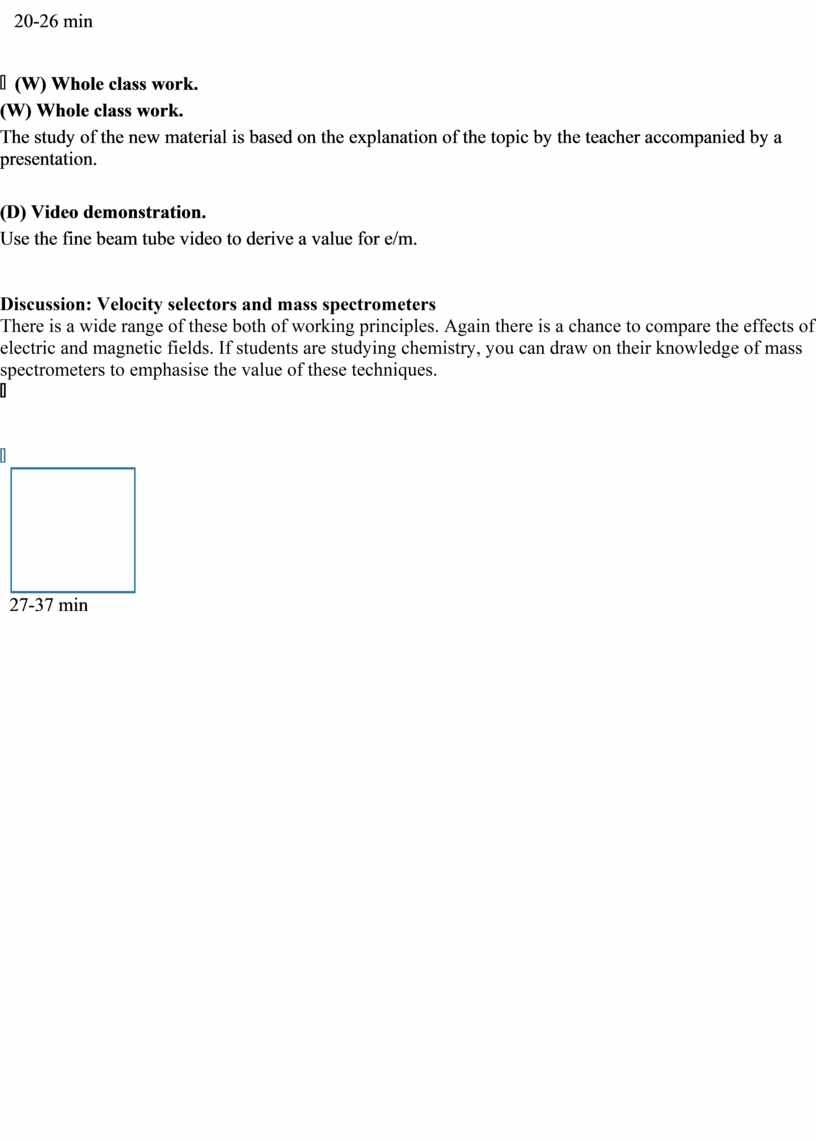Скачать файл